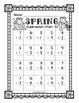# Spring SubtractionSubject
Resource Type
File Type

PDF

(4 MB|22 pages)
Standards
Also included in:
1. This bundle includes:Spring Addition WorksheetsSpring Subtraction WorksheetsSpring Mixed Addition and Subtraction WorksheetsIf you enjoy this resource, follow me to know when other resources and FREEBIES are uploaded. Please rate this product to get TpT credits. This resource is for ONE classroom us
\$7.50
\$6.50
Save \$1.00
2. Do you need some basic subtraction worksheets for your students to use all year? This bundle is jam packed with a total of 198 subtraction worksheets to use all year with your students. Each set contains 9 horizontal and 9 vertical worksheets.Each set includes: 3 horizontal subtraction worksheets fr
\$27.50
\$24.50
Save \$3.00
3. Do you need some basic addition and subtraction worksheets for your students to use all year? This bundle is jam packed with a total of 594 worksheets. You will get 198 addition worksheets, 198 subtraction worksheets and 198 mixed addition and subtraction worksheets to use all year with your student
\$82.50
\$70.00
Save \$12.50
• Product Description
• Standards

The product includes 18 total subtraction worksheets (9 vertical and 9 horizontal). It includes subtraction from to 10, 15, and 20. Each page includes fun Spring clip art. This is a great activity for Kindergarten, 1st Grade, and 2nd Grade students!

Check out the preview to see EVERYTHING that is included.

Other products you may be interested in:

Spring Mixed Addition and Subtraction Worksheets

Time Math Center

Spring Time Worksheets

Spring Writing Paper

Write About a Picture – Spring

Write The Room Easter

Write The Room Easter FREEBIE

Write The Room Spring

Write The Room Spring FREEBIE

If you enjoy this resource, follow me to know when other resources and FREEBIES are uploaded. Please rate this product to get TpT credits. This resource is for ONE classroom use only. Please purchase additional licenses to share it.

Keywords: Subtraction, Spring, Worksheets, Math, 1st Grade, Math Worksheets

Fluently add and subtract within 20 using mental strategies. By end of Grade 2, know from memory all sums of two one-digit numbers.
Add and subtract within 20, demonstrating fluency for addition and subtraction within 10. Use strategies such as counting on; making ten (e.g., 8 + 6 = 8 + 2 + 4 = 10 + 4 = 14); decomposing a number leading to a ten (e.g., 13 - 4 = 13 - 3 - 1 = 10 - 1 = 9); using the relationship between addition and subtraction (e.g., knowing that 8 + 4 = 12, one knows 12 - 8 = 4); and creating equivalent but easier or known sums (e.g., adding 6 + 7 by creating the known equivalent 6 + 6 + 1 = 12 + 1 = 13).
Relate counting to addition and subtraction (e.g., by counting on 2 to add 2).
Understand subtraction as an unknown-addend problem. For example, subtract 10 – 8 by finding the number that makes 10 when added to 8.
Apply properties of operations as strategies to add and subtract. If 8 + 3 = 11 is known, then 3 + 8 = 11 is also known. (Commutative property of addition.) To add 2 + 6 + 4, the second two numbers can be added to make a ten, so 2 + 6 + 4 = 2 + 10 = 12. (Associative property of addition.)
Total Pages
22 pages
N/A
Teaching Duration
N/A
Report this Resource to TpT
Reported resources will be reviewed by our team. Report this resource to let us know if this resource violates TpT’s content guidelines.